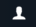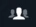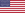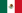# Assault Rigs

Assault Rigs
Basic Information
Type(s)
Video Game
Psygnosis, Perfect Entertainment
Psygnosis
Action
MS-DOS, PlayStation and Saturn
Retail FeaturesPlay Information
1-2North American Release Date(s)
PlayStation
February 11996
Awards | Changelog | Cheats | Codes
Codex | Compatibility | Covers | Credits | DLC | Help
Localization | Manifest | Modding | Patches | Ratings
Reviews | Screenshots | Soundtrack
Videos | Walkthrough
Achievements
GOG | In-Game | Origin | PlayStation Trophies | Retro
Steam | Xbox Live

Assault Rigs is an Action game by Psygnosis, released in 1995 for PC, PlayStation and Sega Saturn. The game is based in the future, where real sport has been overtaken in favour of virtual sport. And everyone's favourite is Assault Rigs. The game runs through like a tank simulator, set inside a 3D virtual environment.

The goal of the single player game is to collect all of the gems in a level, while destroying or avoiding enemies, ultimately reaching the end exit. There are 42 Levels (Plus 15 levels for Playstation two player) featuring jump ramps, elevators, sky ways, trenches, movable blocks and push rods. There are also 20 weapon power-ups, viruses and Dolby Surround sound.

Assault Rigs also has the capability to utilize the Playstation Link Cable, enabling two players with two playstations to play head-to-head with full screens. A unique feature of the Serial link cable usage is that there are 15 additional levels that are only available for play using the cable.

## Level Order (PC and Playstation 1 Versions)

Wel come, Nextgen, This Way, Joy Joy, Noddy, Wastelands, Vertigo, Gem Tower, Bridge, Obliterate, Arena, P B M, Ramps, Oasis, Halls, Coaster, Mine, Look Up, Deadline, Fort, Stairway, Park A Lot, Zamcam, Shoot Me, Wild, Oil Rig, Right Way, Waste Two, Dodge, Air, Jump, Room 101, Firepower, Wave, Push Off, Perimeter, Spiral, Bounce, Castle, Fortress, Lifts Ahoy, Push Me.

### Sega Saturn Version

In the Saturn version of Assault Rigs, all of the levels were the same as the Playstation 1 and PC versions physically but they were given different names. For example, the 1st level is called Practice and the 2nd is called Four Rooms on the Sega Saturn version.

## Levels Numbered with Playstation 1 codes

• Level 01-Wel come-Circle, Circle, Circle, Circle, Circle, Circle
• Level 02-Nextgen-Square, X, Square, X, Triangle, Square
• Level 03-This Way-Triangle, Square, Square, Circle, Circle, Triangle
• Level 04-Joy Joy-Triangle, Square, Triangle, Triangle, Circle, Triangle
• Level 05-Noddy-Square, Triangle, Triangle, Triangle, X, Triangle
• Level 06-Wastelands-Triangle, Square, Circle, Circle, X, Square
• Level 07-Vertigo-X, Square, Square, Square, Circle, Triangle
• Level 08-Gem Tower-Triangle, Square, X, Square, Triangle, Triangle
• Level 09-Bridge-Square, Triangle, Square, X, Triangle, X
• Level 10-Obliterate-Triangle, Triangle, Circle, Square, X, Square
• Level 11-Arena-Triangle, Triangle, X, Triangle, Circle, Square
• Level 12-P B M-Circle, Square, Triangle, Triangle, Triangle, Circle
• Level 13-Ramps-Triangle, Square, Circle, X, Triangle, Square
• Level 14-Oasis-Triangle, Triangle, X, Square, Square, X
• Level 15-Halls-Circle, X, Triangle, Triangle, Triangle, Triangle
• Level 16-Coaster-Circle, Square, Circle, Circle, Circle, Square
• Level 17-Mine-Triangle, Triangle, Triangle, Circle, Triangle, Square
• Level 18-Look Up-Square, Circle, Square, X, Square, Triangle
• Level 19-Deadline-X, X, Square, X, Triangle, Circle
• Level 20-Fort-X, Square, X, Triangle, Triangle, Square
• Level 21-Stairway-Triangle, Square, Triangle, Square, Triangle, Triangle
• Level 22-Park A Lot-Square, Square, Triangle, Circle, Square, Triangle
• Level 23-Zamcam-Circle, X, X, X, X, Triangle
• Level 24-Shoot Me-Triangle, Square, Square, Triangle, Triangle, Triangle
• Level 25-Wild-Triangle, Circle, Triangle, Triangle, Circle, Square
• Level 26-Oil Rig-Square, Circle, Circle, X, Square, X
• Level 27-Right Way-X, Circle, Square, Triangle, Triangle, Square
• Level 28-Waste Two-Square, Circle, Square, Square, Square, Square
• Level 29-Dodge-Triangle, Square, Circle, X, Circle, Circle
• Level 30-Air-Square, Circle, X, Circle, X, Square
• Level 31-Jump-Circle, Square, X, Triangle, Circle, Triangle
• Level 32-Room 101-Triangle, Square, X, Circle, Square, X
• Level 33-Firepower-X, X, Triangle, X, X, Square
• Level 34-Wave-X, Circle, Square, Circle, Circle, Square
• Level 35-Push Off-Circle, Triangle, X, Triangle, X, Triangle
• Level 36-Perimeter-X, Triangle, Triangle, X, X, Square
• Level 37-Spiral-X, Triangle, Triangle, X, Triangle, Square
• Level 38-Bounce-Square, Triangle, Square, Triangle, Square, X
• Level 39-Castle-Square, X, Triangle, X, X, Triangle
• Level 40-Fortress-Triangle, X, Triangle, Circle, Square, X
• Level 41-Lifts Ahoy-Square, X, Triangle, Circle, Triangle, Square
• Final Level-Push Me-Circle, X, Triangle, Circle, Triangle, Square

## Weapons

Weapons appear in floating blue cubes. They are opened by shooting them once. These cubes might also contain Power-ups. Here is a list of weapons:

• Standard ammo - You have an unlimited supply of this ammo.
• Bounce ammo - This ammo comes in rounds of 30 and bounces off walls. Can bounce back and damage yourself!
• Shattershells - Comes in rounds of 25 and bounces off walls. Shatters into 5 separate bullets after they have hit the wall.
• Homing Missiles - Comes in rounds of 6. Homes in on closet enemy.
• Guided Missiles - Comes in rounds of 3. After shooting, you gain control of missile and guide it where you want.
• Laser - Comes in rounds of 15. Like a more powerful version of standard ammo. Shoots long distances.
• Mini Gun - Comes in rounds of 250. Like a quick fire version of standard ammo. Powerful at short distances. Doesn't reach far.

## Power-ups

Power-ups appear in floating blue cubes. They are opened by shooting them once. These cubes might also contain Weapons. Here is a list of Power-ups:

• Drop And Forget Mines - Like ordinary mines but you lay them.
• Shield Deck - After activated, a red shield will appear around your rig. This goes after a short period of time.
• Warp Deck - After activated, you are taken to a random place in the arena.
• Push Block Deck - Disguises you as a push block so enemies ignore you. If you shoot, this block disappears.
• Auto Turret - Only works with rigs that have a movable turret. The turret will point at the direction of the closet enemy. Stops after a certain period of time.
• Sentry Gun Pods - Once dropped from the back of your rig, they become active after a few seconds. They shoot at anything that comes in range, so that includes your rig.

## Game Screen

• 1) Shield Indicator. If one indicator turns black, you explode.
• 2) This is where your power-up and ammunation left is shown.
• 3) Gems collected/Gems needed.
• 4) Time left to complete the level.
• 5) Score.
• 6) Weapon selector. The current weapon has a little red rectangle above its' icon.